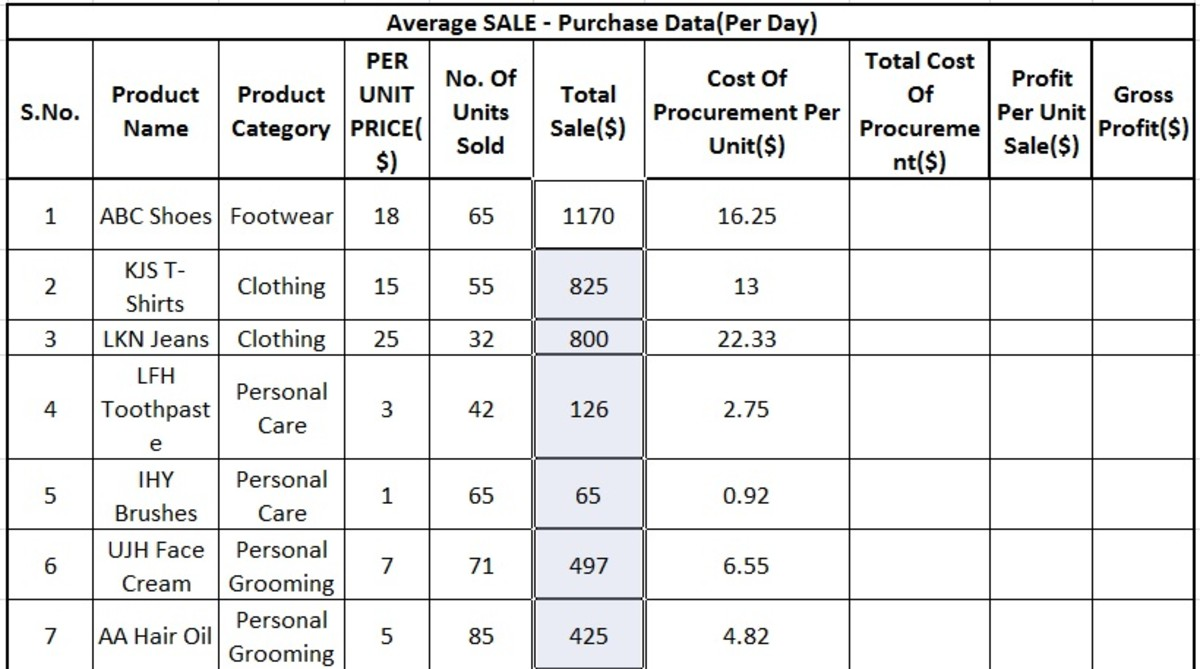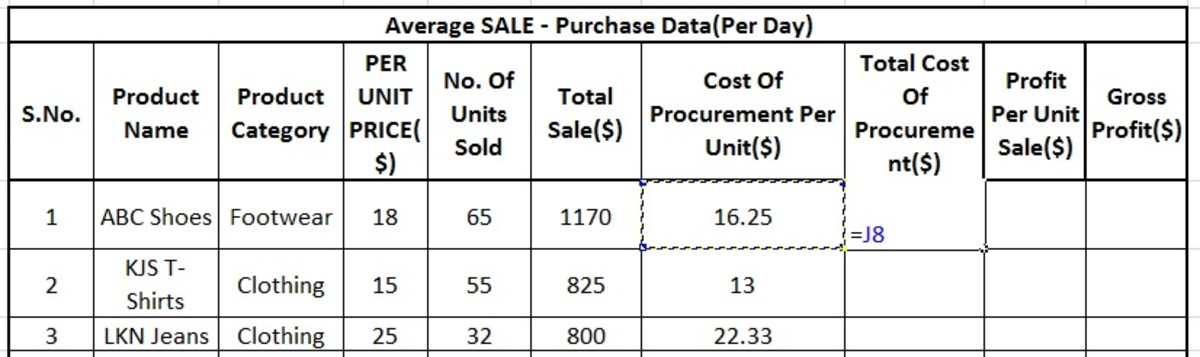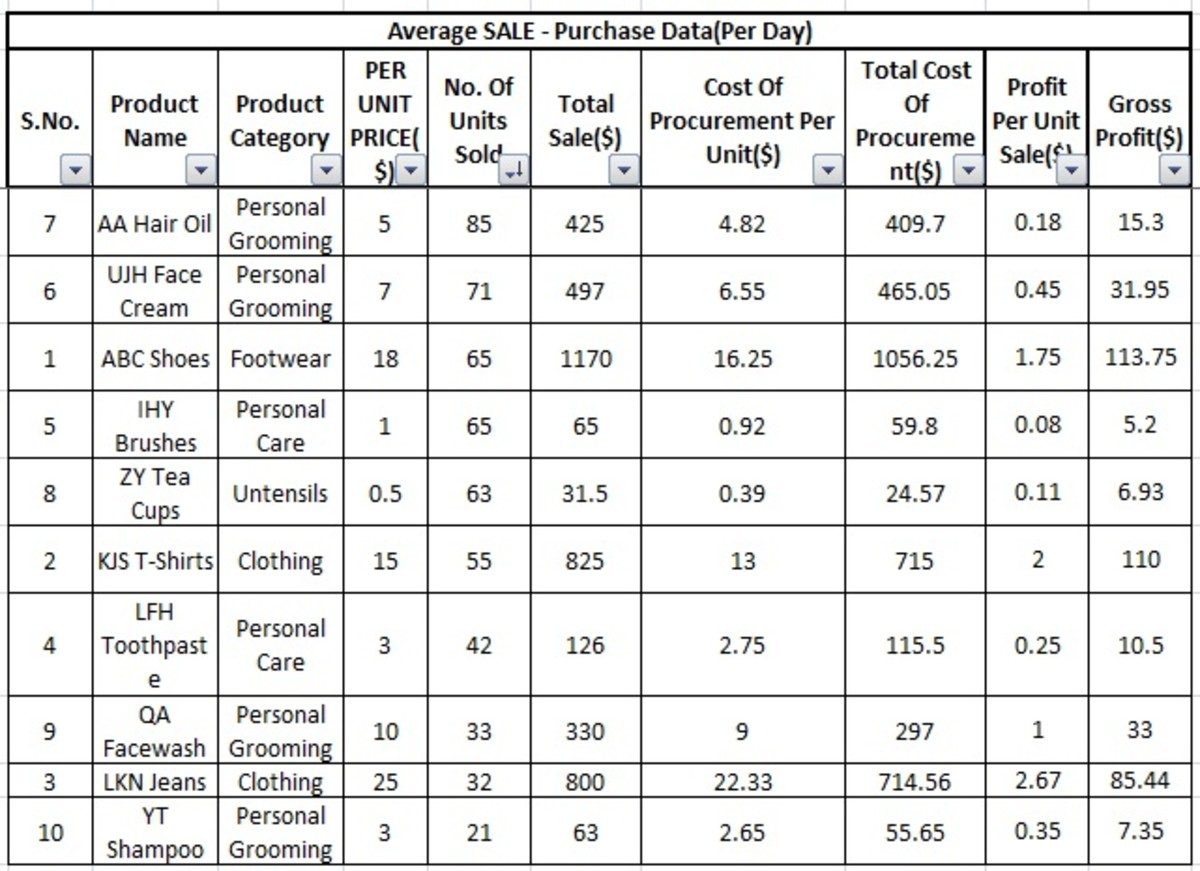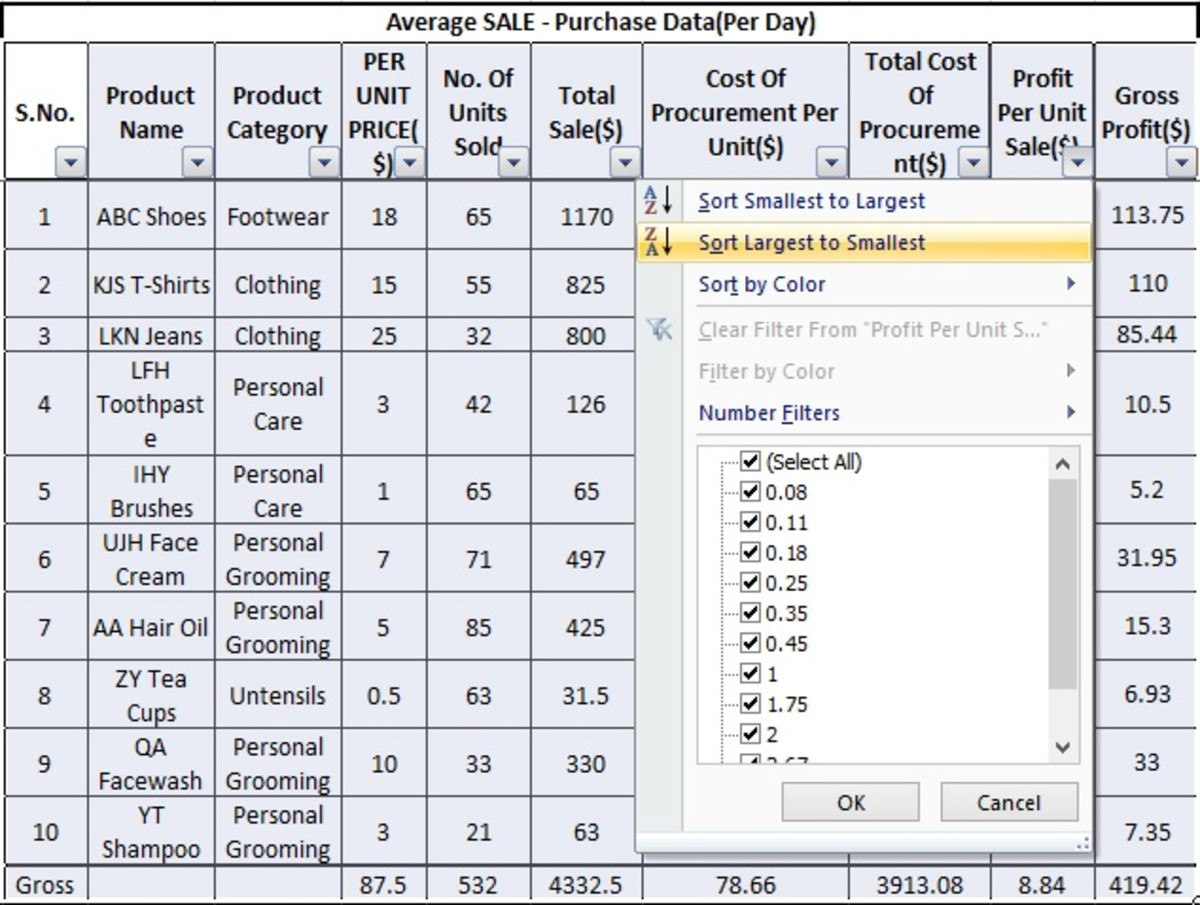# How to Perform the Sales Analysis in Excel to Find Out The Sales Trends

The data analysis is a very important thing in our life. It greatly influences all kinds of professions. An engineer needs to analyze the engineering drawings, reports and technical data for making the most efficient things, a doctor needs to analyze the medical reports of his patients for giving the best diagnosis and when it comes to the businesses the thing becomes more critical. They have to continuously work on data analysis of market trends, profit and costs for making their business stable.

In my opinion, Microsoft Excel in one of the best tools for such type of data analysis. I am going to take the sales purchase data of a departmental store as a reference to show you how you can calculate the profit as well as market trends using Excel.

Here, we have an excel file containing sales and purchase data of a departmental store on an average day. The values provided by the sales and purchase team for different product segments are:-

• Per unit price of each item
• Number of units sold in the day
• Cost of procurement of each unit item that the store has to bear

We will have to analyze this data for the calculation of individual segment profit and then the trends in the market that are profitable for the store. We know that the basic formula for profit calculation is:

Profit = Sales - Purchase

Therefore, we will proceed first by calculating the total sale and then the procurement costs to find out the profit which will be then analyzed to map out the trends.

• First, we will calculate the total sales of each segment by multiplying the per unit sale price with the total number of goods sold in the concerned segment. For this, type "=" sign in the cell shown under total sale column of the first row as shown in the figure.
• Select the corresponding cell under the "per unit price" column. Its borderline will start blinking now.
• In Microsoft Excel, the symbol * represents the multiplication so we will type this symbol.
• Now select the corresponding cell of "number of units sold". It will also start blinking like the earlier one. The resulting cell will show the name of both cells and a multiplication symbol joining them.
• After this, we will press enter and the multiplication value of Unit sale prices cell and Number of units sold cell will be returned in the resulting cell.
• Now we will select this first cell under Total sale(\$) column and drag the selection till the last one(refer picture given below)
Scroll to Continue
• This will make each cell in this column reflecting the product value of corresponding "Number of units sold" column and "Per unit price" column cells. (i.e The formula of Total sale = Per unit price x No. of units sold, will be automatically applied on each row)You can see in this image that all the cells in selected column are reflecting the product value of the two cells on left sideAuthor of this hub

We have calculated the earning from totals sales of each product. In order to figure out the profit, we will have to calculate the cost of bringing these goods to the customers. We have been provided with the cost of procurement of single item in each segment so we will calculate the total cost of procurement of all items with the formula:-

Total cost of procurement = (Cost of procurement per unit) X (No. of units sold)

i.e by multiplying the number of units sold with the cost of procurement of single item for each row(product segment). Here we will proceed as follows:

• In the first cell under the total cost of procurement column, we will type the "=" symbol and then select the first cell under "Cost of procurement per unit" column similarly as we have done in the previous step.The name of the selected cell J8(Blue text) in this image represents its Column number "J" for its horizontal position and Row number "8" for its vertical position in the entire spreadsheetAuthor of this hub
• Now we will type the multiplication symbol * and then select the other cell containing the value of no. of units of the goods sold in the specific segment.
• After pressing the Enter key, the value of the product of both the selected cells will be displayed.
• For applying the same formula on all the cells under this column we will select the cell and drag down similarly as we did previously. The corresponding product values will be displayed for each row.

We have calculated the total sale value and the procurement cost of all the sold items. Now we will get the estimate about what profit is the sale giving to the store from both the individual item and the gross. So as per the relation of (Profit= Sale - Cost), we will apply formulas in the spreadsheet.

• To apply the profit formula we will type = in the concerned cell(Profit per unit sale) and then select the corresponding cell in the "Per unit price" column. This cell will start blinking as shown in the image given below.
• The excel subtraction is similar to the mathematical subtraction formula; the only difference is that the later one is applied from numeric value to numeric value while the first one can be done from cell to cell. Now we will type the subtraction symbol -
• We have the sale amount behind per unit item in hand with the Excel spreadsheet and we have to subtract the cost of procurement of single item from the same to measure the profit gained by the store. In the case of our Excel file, we can do this by selecting the cell containing the cost of procurement per unit sale so we will select the first cell in the "Cost of procurement per unit" column.
• We have created the required equation and now just by pressing the "Enter" key on the keyboard, we will have the resulting value.

While applying the subtraction formula for the calculation of profit in Excel, the cell containing sale value must be selected first and then the cell of the cost of procurement column

• The subtraction formula has been applied to a single cell. Now we will select and drag it to apply the formula on the entire column. The selected cells will start blinking now.
• The blinking cells will return the resulting value as we will release the selection button of the Mouse.

We have calculated the profit from the sale of a single item from each segment and now we have to calculate the gross profit from all the sales of goods in each segment. For this, the values of "Per unit sale price" and "Cost of procurement per unit" used in the previous step will be substituted by "Total sale" and "Total cost of procurement" respectively. Hence the formula to be used will be:-

(Gross profit = Total sale - Total cost of procurement)

• For applying the above formula, we will type the = symbol in the first cell of Gross profit column and then select the corresponding cell under the Total sale column
• The total sale value has been selected and the subtraction symbol - will be added to apply the subtraction formula.
• To complete the equation of the formula, we will select the corresponding cell containing the total cost of procurement.
• Now it is the time for our final step to complete the formula task by just pressing the enter key and the result will be disclosed by the Excel spreadsheet.
• This formula will be applied on the entire column by selecting and dragging this cell similarly as we have done in the previous formulas.
• After releasing the selection button of the mouse, the gross profit value will be automatically filled in all the cells according to the value of total sale and cost of procurement in their respective rows.

We will now calculate the overall sale, procurement cost and profit data of the departmental store. This will include all sales and purchase costs as well as the gross profit of all segment goods combined. It could be done by adding all the values of the cells in their respective columns.

So how we will do this with Excel formulas? Let's have a look now

• At the bottom of the last cell in the first column we will type =SUM( then select the cell just above it.
• Now we will press and hold the ctrl+shift key on the keyboard and then the arrow ↑. By pressing these keys the Excel will select the entire column.
• When all the cells in the specific column have been selected, we will close the bracket with the symbol ) and press the enter key on the keyboard. The total value of the number of units sold will be displayed in the formulated cell.
• To apply this summation formula on all the cells in the last row(With text Gross in the previous image), we will copy(with ctrl+c) the cell(with value 87.5 shown in the previous image) and paste in all the cells in its row. These cells will automatically reflect the summation of all the cells in their respective columns. It is because the formula can be directly copied from cell to cell.

So finally we have compiled the raw data required our analysis purpose. We will now use the filter tool to find out the most profitable deals.

First of all, we will select all the cells by pressing(CTRL+A).

• Now the filters will be applied on all the columns by pressing alt+D+F+F key combination in a sequence on the keyboard. After pressing the filter shortcut, the triangle symbol of filters will appear on the top row with indexes as shown in the image given below.
• The first thing we will analyse now is the number of goods sold in each product segment and then we will be able to finalize that by sale size which product is performing the best of all. To apply this filter, we will click on the triangle symbol being shown on the cell with the description, "No. Of units sold"
• The items will be sorted from largest to smallest(text shown in the previous image) as it will make the higher number of sales being shown on the top and the lower ones at the bottom.Number of goods sorted from largest to smallestAuthor of this hub

From this image we can point out the following conclusions:

• The "AA Hair oil" is selling the most with 85 quantities sold in the day.
• The lowest sale occurred from YT shampoo with 21 items sold in the day.
• Category wise, the personal grooming products remained on the top while the items of clothing and utensils remained low in the sale scenario.

The next thing that a wise businessman will analyze from the above values, is the individual item profit from the sale of the goods from each segment. It will give them an idea about 'For Which Product They Are Getting The Largest Margin" and they may try to persuade their sales and marketing staff to give more emphasis on selling these products.

• For applying a filter on the profit of each unit in our excel spreadsheet we will repeat the previous step but this time on the "Profit per unit sale" column.Applying filter on the profit per unit sales columnAuthor of this hub

So we have applied the filter on "Profit Per unit sales" column showing profit margin in descending order from top to bottom. After careful analysis of this column, we can conclude the following points:

• The largest profit margin is from the sale of LKN jeans.
• The IHY brushes remained on the bottom of this column for being the least profitable item in terms of single unit sales.
• The Clothing and Footwear segment is giving more profit per unit sale.

After getting these analysis points the store may decide to increase the stock of clothing and footwear items for increasing the revenue.

The final analysis that may be put on the sheet is for the "Gross Profit". Let's have it now;

So the final filter has given the results as shown in the above image. We can conclude the following points from the analysis:

• The ABS shoes are the most feasible deal in terms of sales quantity and per unit profit margin.
• The IHY brushes though these products remained in high stakes of the number of units sold but lagged behind all the segments while counting the gross profit.

## Conclusion

This was a sample exercise that one can easily perform in the Microsoft Excel to analyze some sales, purchase and profit data. You can apply the same procedure on different types of data to get some fruitful results. To conclude with, I would like to say that the best efforts have been made by myself to complete this exercise and if you think anything has been left unresolved then please let me know in the comments section below, anything away from the subject of this article must be avoided.

Thanks for taking time to read my work, Happy Hubbing!

This content is accurate and true to the best of the author’s knowledge and is not meant to substitute for formal and individualized advice from a qualified professional.